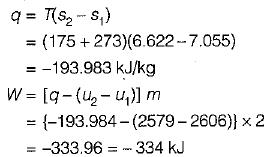Courses

# Test: Work & Heat Transfer - 2

## 20 Questions MCQ Test Topicwise Question Bank for Mechanical Engineering | Test: Work & Heat Transfer - 2

Description
This mock test of Test: Work & Heat Transfer - 2 for Mechanical Engineering helps you for every Mechanical Engineering entrance exam. This contains 20 Multiple Choice Questions for Mechanical Engineering Test: Work & Heat Transfer - 2 (mcq) to study with solutions a complete question bank. The solved questions answers in this Test: Work & Heat Transfer - 2 quiz give you a good mix of easy questions and tough questions. Mechanical Engineering students definitely take this Test: Work & Heat Transfer - 2 exercise for a better result in the exam. You can find other Test: Work & Heat Transfer - 2 extra questions, long questions & short questions for Mechanical Engineering on EduRev as well by searching above.
QUESTION: 1

### Work done is zero for the following process

Solution:

For constant volume process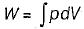Since, dV = 0
W = 0
W = 0
Also for throttling process
W = 0

QUESTION: 2

### In free expansion process

Solution: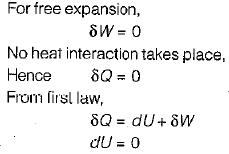QUESTION: 3

### Which one of the following thermodynamic process approximates the steaming of food in a pressure cooker?

Solution:

Since volume of pressure cooker is constant hence it is an Isochoric process.

QUESTION: 4

The cyclic integral of (δQ - δW)  for a process is

Solution:

For a process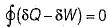QUESTION: 5

Heat transferred to a closed stationary system at constant volume is equal to

Solution: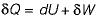For constant volume, close system work = 0
Hence,
δQ = dU

QUESTION: 6

Thermodynamic work is the product of​

Solution: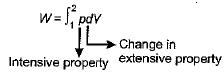QUESTION: 7

The maximum amount of mechanical energy that can be converted into heat in any process

Solution:

Since mechanical energy is high grade energy and heat is low grade energy, 100% conversion of high grade energy into low grade energy is possible.

QUESTION: 8

The expression for work done during a process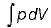is applicable for

Solution:
QUESTION: 9

A paddle wheel used for stirring a liquid contained in a tank supplied 5000 kJ of work and during the stirring operation the tank lost 1500 kJ of heat to the surroundings. If the tank and liquid are considered as a system the change in its internal energy will be

Solution:

From the first law of thermodynamics
δQ = dU + δW
-1500 = dU - 5000
dU = 5000 - 1500
dU = 3500 kJ

QUESTION: 10

The change in enthalpy of a closed system is equal to the heat transferred, if the reversible process takes place at constant

Solution: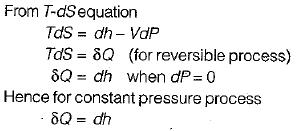QUESTION: 11

​Which one of the following statement holds good for the equation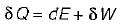Solution: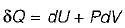This equation holds good for any process reversible or irreversible.

QUESTION: 12

Change in internal energy in a reversible process occurring in a closed system is equal to the heat transferred, if the process occurs at constant

Solution: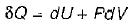for constant volume process dV= 0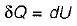QUESTION: 13

300 kJ/s of heat is supplied at a constant fixed temperature of 290°C to a heat engine. The heat rejection takes place at 8.5°C. Then match the following
Results obtained                    Cycle
A. 215 kJ/s are rejected      1. reversible
B. 150 kJ/s are rejected      2. irreversible
C. 75kJ/s are rejected         3. impossible
Codes:
A   B   C
(a) 1   2   3
(b) 2   1   3
(c) 3   2   1

Solution:
QUESTION: 14

Match List-1 with List-11 and select the correct answer using the codes given below.
List - I
A. Work done
B. Thermal equilibrium
C. Internal energy
D. No work and heat interaction
List - II
1. Point function
2. Path function
3. Isolated system
4. Equality of temperature
Codes:
A   B   C   D
(a) 2   4   1   3
(b) 2   3   4   2
(c) 3   1   2   4
(d) 4   2   3   1

Solution: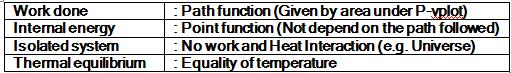QUESTION: 15

A 2 kW electric resistance heater submerged in 5 kg water is turned on and kept on for 10 min. During the process 300 kJ of heat is lost from the water the temperature rise of water is

Solution:

P = 2 kW; Mass - 5 kg
Heat lost = 300 kJ
Heat supplied - 2 x 600 - 1200 kJ
Net heat supplied
= 1200 - 300
= 900 kJ
900 = 5 x 4.18 (ΔT)
ΔT = 43.1°C

QUESTION: 16

One kg of ice at 0°C is completely melted into water at 0°C at 1 bar pressure. The latent heat of fusion of water is 333 kJ/kg and densities of water and ice at 0°C are 999 kg/m3 and 916 kg/m3 respectively. What are the approximate values of work done and energy transferred as heat for the process respectively.

Solution: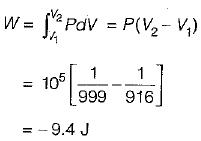Heat transfer = mL
= 1 x 333 = 333 kJ

QUESTION: 17

It is desired to bring about a certain change in the state of a system by performing work on the system under adiabatic conditions.

Solution:
QUESTION: 18

Which one of the following statements is true?

Solution:

The efficiency of a heat engine increases as the temperature of the heat source is increased while keeping the temperature of the heat sink fixed.

QUESTION: 19

Match the following:
A. Heat                       I. State function
B. Internal energy      II. Path function
C. Work
D. Entropy

Solution:

Heat is a path function
Internal energy is a state function
Work is a path function
Entropy is a state function.

QUESTION: 20

2 kg of steam in a piston-cylinder device at 400 kPa and 175°C undergoes a mechanically reversible, isothermal compression to a final pressure such that the steam becomes just saturated. What is the work, W, required for the process?
Data:
T = 175°C;
P = 400 kPa
v = 0.503 m3/kg
u = 2606 kJ/kg
s = 7.055 kJ/kgK
Saturated vapour, v = 0.216 m3/kg
u = 2579 kJ/kg, s = 6.622 kJ/kgK

Solution: